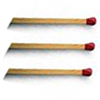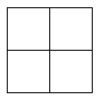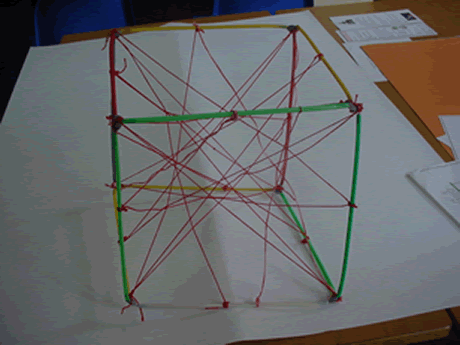#### You may also like### Matchsticks

Reasoning about the number of matches needed to build squares that share their sides.### Little Boxes

How many different cuboids can you make when you use four CDs or DVDs? How about using five, then six?### Two Squared

What happens to the area of a square if you double the length of the sides? Try the same thing with rectangles, diamonds and other shapes. How do the four smaller ones fit into the larger one?

# Construct-o-straws

##### Age 7 to 11Challenge Level

Nico and Daniel from Lower Heath CE Primary School submitted the following solution:

There are 14 ways to go from one vertex to another. Our key was B=back, F=forwards, R=right, L=left, D=down and U=up.

Part 1
1.B,R,D
2.R,B,D
3.D,R,B
4.D,B,R
5.R,D,B
6.B,D,R
7.B,D,F,R,B
8.R,D,L,B,R
9.D,B,U,R,D
10.D,R,U,R,D
11.R,B,L,F,D,R,B
12.R,B,L,F,D,B,R
13.B,R,F,L,D,R,B
14.B,R,F,L,D,B,R

Notice how they worked methodically and recorded their results in a systematic way. Can you see how the results would be different if we were to impose more rules, for instance only being allowed to visit each vertex once? If there is no limit to how many times you can visit each vertex, then there would be plenty more routes that could go on forever! Nico and Daniel also tackled the second half of the problem:

Part 2 Here is a picture of our model:Part 3 As more threads are attached it will be harder to add more threads and it will look more complicated.

Part 4 The threads make a shape like a 3D star.

Part 5 We think that when we spin the cube it will look like there is a cube in the middle. We were right.

Excellent work, thank you for sending it in to us and well done for cracking this tough nut!